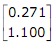# When a program is being executed in an 8085 microprocessor,its program counter contains

1.  the number of instructions in the current program that have already been executed

2.  the total number of instructions in the program being executed

3.  the memory address of the instruction that is being currently executed

4.  the memory address of the instruction that is to be executed next

4

the memory address of the instruction that is to be executed next

Explanation :
No Explanation available for this question

# A control system is defined by the following mathematical relationship .The response of the system as t→  is

1.  x=6

2.  x=2

3.  x=2.4

4.  x=-2

4

x=2.4

Explanation :
No Explanation available for this question

# A lead compensator used for a closed loop controller has the following transfer function .for such a lead compensator

1.  a

2.  b

3.  a>Kb

4.  a

4

a<b

Explanation :
No Explanation available for this question

# A second order system starts with an initial condition of   without any external input.The state transition matrix for the system is given by .The state of the system at the end of 1 second is given by

1.

2.

3.

4.

4Explanation :
No Explanation available for this question

# A manganin swamp resistance is connected in series with a moving coil ammeter consisting of a milli-ammeter and a suitable shunt in order to

1.  minimize the effect of temperature variation

2.  obtain large deflecting torque

3.  reduce the size of the meter

4.  minimize the effect of stray magnetic fields

4

minimize the effect of temperature variation

Explanation :
No Explanation available for this question

# The effect of stray magnetic fields on the actuating torque of a portable instrument is maximum when the operating field of the instrument and the stray fields are

1.  perpendicular

2.  parallel

3.  inclined at 60o

4.  inclined at 30o

4

parallel

Explanation :
No Explanation available for this question

# A reading of 120 is obtained when a standard inductor was connected in the circuit of a Q-meter and the variable capacitor is adjusted to a value of 300 pF.A lossless capacitor of unknown value Cx is then connected in parallel with the variable capacitor and the same reading was obtained when the variable capacitor is readjusted to a value of 200 pF.The value of Cx in pF is

1.  100

2.  200

3.  300

4.  500

4

100

Explanation :
No Explanation available for this question

# Figure shows a thyristor with the standard terminators of anode (A),cathode (K),gate(G) and the different junctions named J1,J2 and J3.when the thyristor is turned on and conducting

1.  J1 and J2 are forward biased and J3 is reverse biased

2.   J1 and J3 are forward biased and J2 is reverse biased

3.  J1 is forward biased and J2 and J3 are reverse biased

4.  J1,J2 and J3 are all forward biased

4

J1 and J3 are forward biased and J2 is reverse biased

Explanation :
No Explanation available for this question

# Figure shown a MOSFET with an integral body diode.It is employed as a power switching device in the ON and OFF states through appropriate control.The ON and OFF states of the switch are given on the VDS-IS plane by

1.  fig.A

2.  fig.B

3.  fig.C

4.  fig.D

4

fig.D

Explanation :
No Explanation available for this question

1.  0.5

2.

3.  (1- )

4.

4